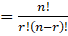# If nC12 = nC8, then n is equal toQuestion:

If nC12 = nC8, then n is equal to
A. 20
B. 12
C. 6
D. 30

Solution:

A. 20

Explanation:

According to the question,

nC12 = nC8

We know that,

nCrn – 8 = 12

n = 20

Hence, Option (A) 20 is the correct answer.